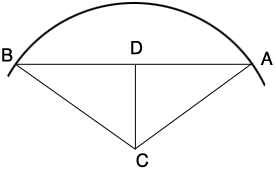SEARCH HOMEMath Central Quandaries & QueriesQuestion from Nishan: If chord length is given along with angle then how to calculate the radius.Hi,

I drew a diagram and labeled some of the points$D$ is the midpoint of $AB$ so you know the length of $DA.$ You know the measure of the angle $BCA$ so you can determine the measure of the angle $DCA.$ Angle $ADC$ is a right angle. Do you see why? What trig functions relates the lengths of the sides $CA$ and $AD$ in triangle $ADC,$ and the measure of the angle $DCA?$

PennyMath Central is supported by the University of Regina and the Imperial Oil Foundation.